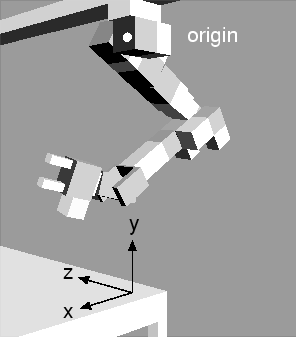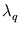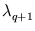Next: 4.5.2 Results Up: 4.5 Kinematic arm model Previous: 4.5 Kinematic arm model

## 4.5.1 Methods

A robot arm with six rotatory degrees of freedom was simulated. It corresponds to the real robot arm in chapter 6. Figure 4.9 shows the setup of the model. By modeling the geometry of the arm and its surrounding, it could be determined if a collision occurred between different parts of the arm or between the arm and the surrounding obstacles. This information was used for the binary collision variable.The training set was generated by randomly choosing 50 000 joint-angle sets (each set contains six angles). Angles were chosen from a uniform interval of ±120 degrees centered at a predefined zero position. For each joint-angle set, the end-effector position was determined from the geometric arm model. It was also calculated if the angle set resulted in a collision. Thus, each training pattern is 10-dimensional and contains six joint angles, three end-effector coordinates, and one collision variable. Only training patterns with an end-effector position inside a workspace of 500×500×500mm above the table were included in the training set. Moreover, the patterns were chosen such that half of the set were collision trials and half no-collision trials. All values were scaled to fit in a 10-dimensional cube with side length one.

The training parameters for NGPCA and NGPCA-const were the same as in section 4.4.1. In most tests (apart from those that did show the dependence on m or q), the local PCA mixture models contained m = 200 units and q = 6 principal components. Neural Gas (Martinetz et al., 1993) was used as an alternative training method (the same training parameters as for NGPCA were used). It was composed of 980 code-book vectors, resulting in the same number of free parameters as used for the mixture model. By setting the potential of the code-book vectors to the Euclidean distance, the same recall algorithm could be used as for the local PCA method. The abstract RNN was further compared to a multi-layer perceptron that had one hidden layer with 200 neurons (smaller or higher numbers did not improve the performance). In the hidden layer, a sigmoid activation function was used. The weights were initialized with random values drawn uniformly from the interval [- 0.5;0.5]. Forward and inverse direction had to be learned separately. In both cases, the network trained 2 000 epochs of resilient propagation (Riedmiller and Braun, 1993).

The local dimensionality of the kinematic manifold is six (the six joint angles are the free parameters), and this guided the choice of the parameter q. In general, however, the local dimensionality of a data distribution is not known a priori. To obtain the number of dimensions, the following algorithm was used.

For every data point, the 30 closest (Euclidean distance) neighbors were selected. Then, on each neighborhood a PCA was carried out. The resulting eigenvalues were averaged over all data points. The size of successive averaged eigenvaluesis expected to decrease slowly until the local dimensionality is reached, and then, this size breaks down and reaches a noise level. Thus, the local dimensionality can be obtained as the position of the first peak in the series/(Philipona et al., 2003).

The trained abstract RNN could recall in both inverse and forward directions. For the inverse direction, the end-effector coordinates and the collision state are the offset of a constrained space, and the recall algorithm had to find the joint angles. Position errors were calculated between the desired end-effector coordinates and the ones obtained by feeding the recalled joint angles into the geometric arm model. Collision errors were obtained in a similar way. Desired end-effector coordinates were taken from a 11×11×11 grid inside the working space.

In the forward direction, the six joint angles were given, and the network had to find the end-effector coordinates and the collision state. The position error and collision-prediction error were computed by directly comparing the network output with the result from the geometrical model. The test pattern set used here was randomly generated in the same way as the training set. It contained 1331 patterns (the same number as for the inverse direction).

Two further tests show how the abstract RNN copes with additional noise dimensions and how the recall results depend on the dimensionality of the input. For the first of these tests, another training set was generated with three additional noise dimensions. In this set, each training pattern had three additional variables, whose values were randomly drawn from the interval [- 1.0;1.0].

To investigate the effect of the number of input dimensions on the performance, errors were computed for arbitrary recall directions. In a test with arbitrary directions that include one-to-many mappings, as in the case of the inverse direction, a recalled pattern cannot be directly compared with a test pattern. Moreover, no function exists that could map the output back onto the input (to compute the error as above). As a solution, the square error was computed as the minimal squared distance to the manifold given by the geometric arm model. This optimization (in the subspace of joint-angles) was carried out with the `Downhill Simplex Method' (Press et al., 1993, p. 408). The square error was computed on all test patterns for each possible number of input dimensions, while for each test pattern, the single input dimensions were chosen at random. This random choice avoids geometric effects that might favor specific directions.Next: 4.5.2 Results Up: 4.5 Kinematic arm model Previous: 4.5 Kinematic arm model
Heiko Hoffmann
2005-03-22